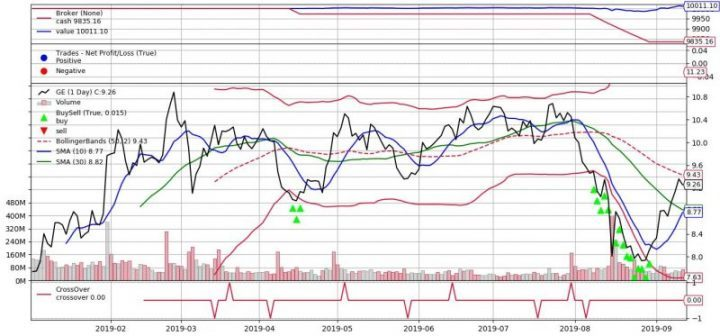In the post Bitcoin trading with Python — Bollinger Bands strategy analysis the author reported 34% returns over the initial investment on back test. Does it work all the time? Is it the best strategy?

Let us use backtrader platform and compare several strategies just for buy signal. Backtrader is a feature-rich Python framework for backtesting and trading. Backtrader allows you to focus on writing reusable trading strategies, indicators and analyzers instead of having to spend time building infrastructure.

We will use the following strategies:

Crossover – based on two simple moving averages (SMA) with periods 10 and 30 days. Buy if fast SMA line crosses slow to the upside.

Consecutive 2 prices (Simple1) – Buy if two consecutive prices are decreasing – if current price is less than previous price and previous price is also less than previous price

```  if self.dataclose < self.dataclose[-1]:
if self.dataclose[-1] < self.dataclose[-2]:
```

Consecutive 4 prices (Simple2) – Buy if within 4 price windows we have decrease more than 5% of original price between any two prices from this window:

```   if tr_str == "simple2":
# since there is no order pending, are we in the market?
if not self.position: # not in the market
if (self.dataclose - self.dataclose[-1]) < -0.05*self.dataclose or (self.dataclose - self.dataclose[-2]) < -0.05*self.dataclose or (self.dataclose - self.dataclose[-3]) < -0.05*self.dataclose or (self.dataclose - self.dataclose[-4]) < -0.05*self.dataclose:
#if self.dataclose[-1] < self.dataclose[-2]:
```

Bollinger Bands – Buy when the price cross the bottom band.

```  if self.data.close < self.boll.lines.bot:
```

Our starting asset value is 10000. We use daily prices.
After running above strategies we get results like below:Final Vaues for Strategies

```cross 9999.06
simple1 9999.31
simple2 9999.91
BB 10011.099999999999
```

Thus we see that Bollinger Band looks more promising comparing with other strategies that we tried. However we did not do any optimization – using different parameters to improve performance of strategy. We learned how to set different strategies with backtrader and got understanding how to issue buy signal. Below you can find full source code.

```# -*- coding: utf-8 -*-
import matplotlib
import matplotlib.pyplot as plt

from datetime import datetime

matplotlib.use('Qt5Agg')
plt.switch_backend('Qt5Agg')

# Create a subclass of Strategy to define the indicators and logic
class SmaCross(bt.Strategy):
# parameters which are configurable for the strategy
params = dict(
pfast=10,  # period for the fast moving average
pslow=30,   # period for the slow moving average
)
params['tr_strategy'] = None

def __init__(self):

self.boll = bt.indicators.BollingerBands(period=50, devfactor=2)
self.dataclose= self.datas.close    # Keep a reference to
self.sma1 = bt.ind.SMA(period=self.p.pfast)  # fast moving average
self.sma2 = bt.ind.SMA(period=self.p.pslow)  # slow moving average
self.crossover = bt.ind.CrossOver(self.sma1, self.sma2)  # crossover signal
self.tr_strategy = self.params.tr_strategy

def next(self, strategy_type=""):
tr_str = self.tr_strategy
print (self.tr_strategy)

# Log the closing prices of the series
self.log("Close, {0:8.2f} ".format(self.dataclose))
self.log('sma1, {0:8.2f}'.format(self.sma1))

if tr_str == "cross":
if not self.position:  # not in the market
if self.crossover > 0:  # if fast crosses slow to the upside

if tr_str == "simple1":

if not self.position: # not in the market
if self.dataclose < self.dataclose[-1]:
if self.dataclose[-1] < self.dataclose[-2]:

if tr_str == "simple2":

if not self.position: # not in the market
if (self.dataclose - self.dataclose[-1]) < -0.05*self.dataclose or (self.dataclose - self.dataclose[-2]) < -0.05*self.dataclose or (self.dataclose - self.dataclose[-3]) < -0.05*self.dataclose or (self.dataclose - self.dataclose[-4]) < -0.05*self.dataclose:

if tr_str == "BB":
#if self.data.close > self.boll.lines.top:
#self.sell(exectype=bt.Order.Stop, price=self.boll.lines.top, size=self.p.size)
if self.data.close < self.boll.lines.bot:

print('Current Portfolio Value: %.2f' % cerebro.broker.getvalue())

def log(self, txt, dt=None):
# Logging function for the strategy.  'txt' is the statement and 'dt' can be used to specify a specific datetime
dt = dt or self.datas.datetime.date(0)
print('{0},{1}'.format(dt.isoformat(),txt))

return

self.log('OPERATION PROFIT, GROSS {0:8.2f}, NET {1:8.2f}'.format(

strategy_final_values=[0,0,0,0]
strategies = ["cross", "simple1", "simple2", "BB"]

for tr_strategy in strategies:

cerebro = bt.Cerebro()  # create a "Cerebro" engine instance

data = bt.feeds.GenericCSVData(
dataname='GE.csv',

fromdate=datetime(2019, 1, 1),
todate=datetime(2019, 9, 13),

nullvalue=0.0,

dtformat=('%Y-%m-%d'),

datetime=0,
high=2,
low=3,
open=1,
close=4,
volume=6,
openinterest=-1

)

print ("data")
print (data )

# Print out the starting conditions
print('Starting Portfolio Value: %.2f' % cerebro.broker.getvalue())

result=cerebro.run()  # run it all
figure=cerebro.plot(iplot=False)
figure.savefig('example.png')

# Print out the final result
print('Final Portfolio Value: %.2f' % cerebro.broker.getvalue())
ind=strategies.index(tr_strategy)
strategy_final_values[ind] = cerebro.broker.getvalue()

print ("Final Vaues for Strategies")
for tr_strategy in strategies:
ind=strategies.index(tr_strategy)
print ("{} {}  ". format(tr_strategy, strategy_final_values[ind]))

```